# Lecture 30: The Expanding Universe

## Matter content of the Universe

### What do we have in our Universe at the present time ?

• We can see luminous matter . We can count stars in our galaxy, then count galaxies to get an estimate (but there are other better, although indirect ways). We get density of luminous matter &rhob (this is Greek letter "rho", b stands conventionally for "baryons" - protons and neutrons)
&rhob = 4.2 x 10-28 kg/m3
This is equal to 1.5 protons per cubic meter.
• Presence of the dark matter reveals that there is other gravitating matter that we do not see. Current estimates for the total matter density &rhom
&rhom = 2.4 x 10-27 kg/m3
i.e we see 17% of the total matter. Error of modern determination is in single percents.
• There is Cosmic Microwave Background radiation. How much energy is in it ? This can be determined very accurately. We express is as mass density of radiation &rhorad (mass=E/c2)
&rhorad = 4 &sigma T4/c3= 4.6 x 10 -31 kg/m3

### Is is a lot or a little ?

• Need to compare with something cosmological.
• There is a critical density &rhoc that is associated with the expansion rate (Hubble constant) H0
&rhoc = 3 H02/(8 &pi G) = 1 x 10 -26 kg/m3 for H0=73 km/s/Mpc
• So we have the following ratios to the critical density
&Omegarad = &rhorad / &rhoc = 4.6 x 10 -5  ,  &Omegab = &rhob / &rhoc = 0.042  ,  &Omegam = &rhom / &rhoc = 0.24
• We also define total mass density ratio

&Omega0 = &Sigma &Omegai (sum of all contributions)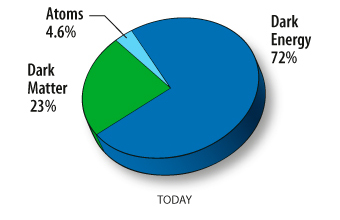## Matter content and Spatial Geometry

• There are also three types of geometries the Universe could have.
• The three types of geometries are related to &Omega0
• One of the goals of observational cosmology is to determine the geometry of the universe.
 If the combined density of the universe today is greater than the critical density, &Omega0 > 1 , then the universe has a closed geometry. If the universe were two-dimensional, its geometry would be like the surface of a sphere.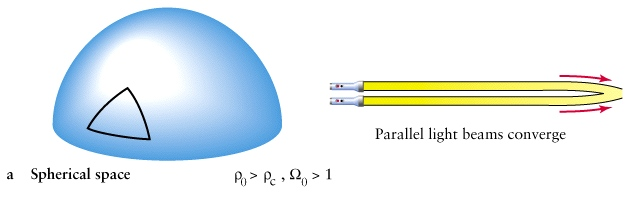If the density of the universe (today) is smaller than the critical density, &Omega0 < 1 , then the universe is open. If it were two-dimensional would have the geometry of a hyperboloid, which is saddle-shaped.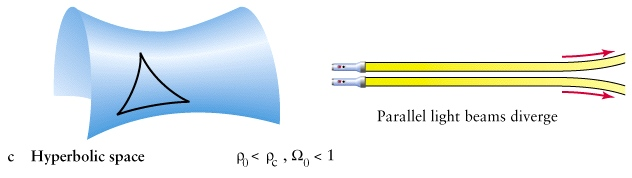If the density is exactly the critical density, &Omega0 = 1 , then has flat space. the universe is the critical solution. The geometry of the critical solution is flat, so that distances between points can be calculated using the Pythagorean Theorem.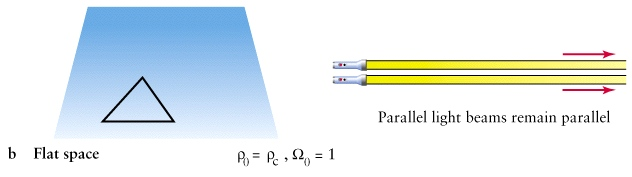## So what about our Universe ?

• One should not think that density causes the space to adopt a geometry. Remember critical density depends on expansion rate. Sop it is more like if the Universe has a given geometry and a matter content, it will expand with the rate to reflect it.
• So we have &Omegarad+&Omegam=0.24. Does it mean our space is open with hyperbolic geometry ? Only if we are sure that we accounted for all possible kinds of matter and that Einstein equations are correct.
• However, one can measure the curvature of the space independently, and more accurately that accounting for different types of matter.
• You need a standard rod (something with known physical size) at a known distance . Distances better be large, cosmological !
• Then measuring its angular diameter and comparing with small angle formula, one can find the geometry of space. Small angle formula is valid only if space is flat !
• We shall discuss tomorrow how CMB can provide such standard rod. The conclusion is
&Omega0 = 1.00-1.02
i.e our space is flat !
• So where is the 75% of density ? There must be some other invisible matter (or modification to Einstein equations). It is known under the name of cosmological constant , &Lambda -term, dark energy ...

## Evolution of density throughout the time

• As Universe expands, the density of matter changes
• We say "the expansion of the universe is dominated by &Lambda term", or "universe is radiation dominated", which specifies what component has the largest contribution to the total density
• At the present time, according to the accepted model, the Universe is dark energy dominated
 However, the density of different types of matter changes differently as Universe expands Ordinary matter. Its density changes because the volume of the Universe changes, while no new matter is created. &rhom ~ 1/V ~ 1/a3 where "a" is the distance scale between galaxies. Therefore with redshift &rhom/&rhom(t0)= a(t0)3/a3 = (1+z)3 Radiation. Best to think about photons. Its number density changes ~ 1/V as well, whoever each photon wavelength is redshifted, so energy of individiual photon also changes as E ~ (1+z) ~ 1/a. Therefore the energy density (expressed as mass density) of radiation evolves as &rhom/&rhom(t0)= a(t0)3/a3 x a(t0)/a = (1+z)4 Dark energy - its density does not change at all, that's why it is called cosmological constant ! &rho&Lambda = const Therefore in the past the balance between the different types of matter was different. The Universe passes through radiation-dominated to matter-dominated to cosmological constant-dominated stages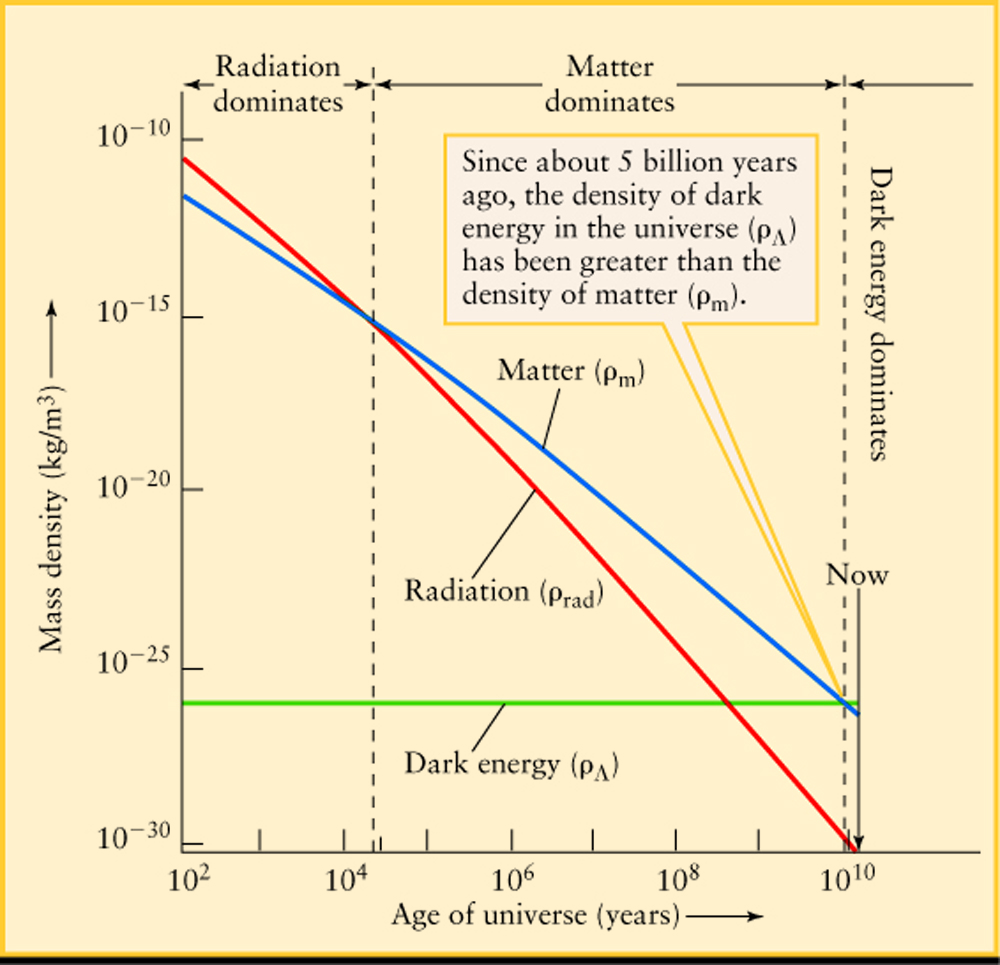## Redshift as look back time - a reminder

• In cosmology the light signals were emitted in the past and are observed by astronomer now.
• Earlier the light signal was emitted, more it is redshifted by the time it reaches us.
• Thus, the redshift z of the source is the measure how far in the past the light was emitted.
• Higher 'z' - further in the past the source is (and also - further away).
• z=0 is the present moment (the light is emitted right here, and took no time to travel, so photons di not change their wavelength at all).
• z=infinity at the start of Big Bang, when we consider t=0
• Relation between redshift and time depends on how exactly the expansion of the Universe proceeded.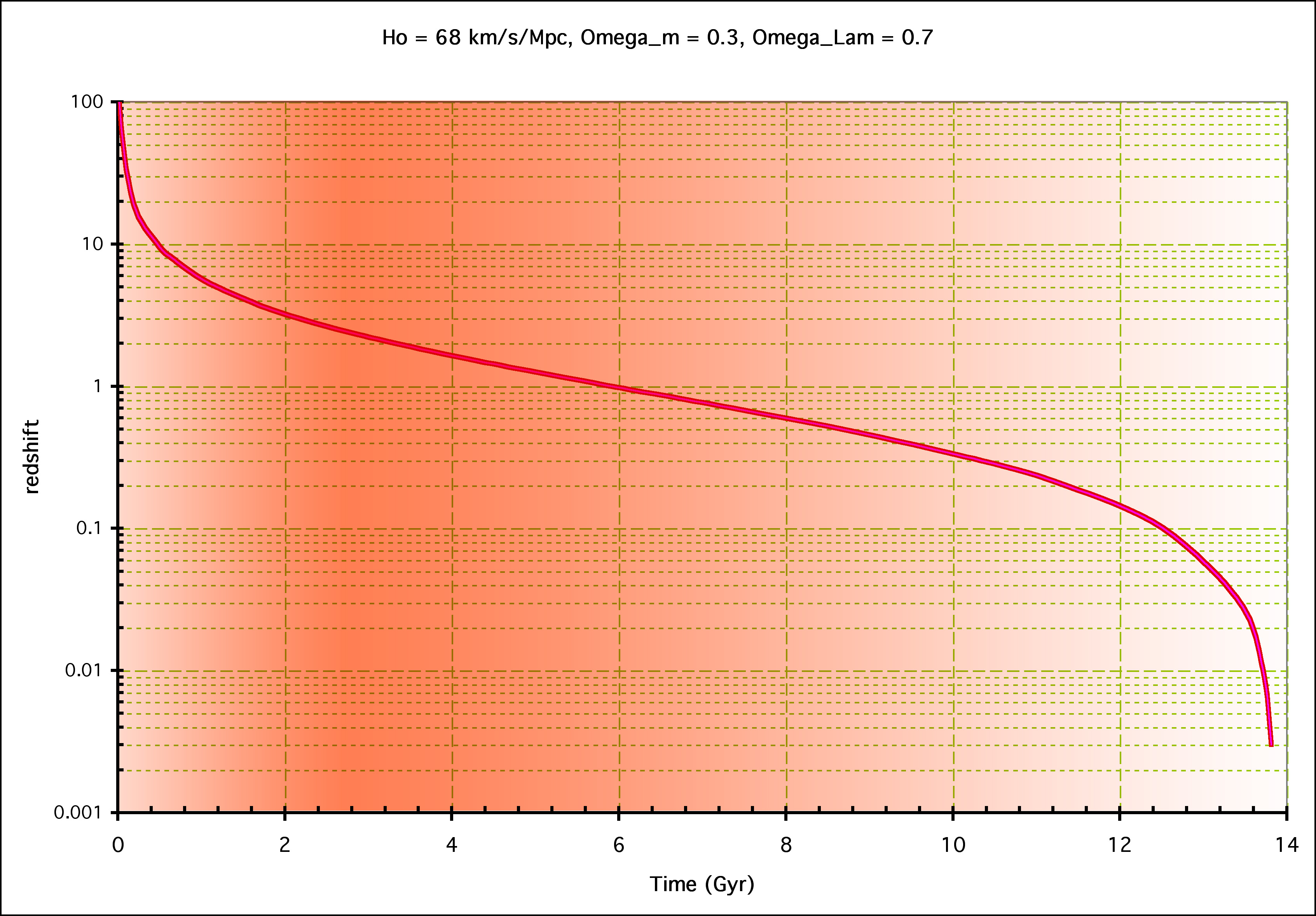## Changes in the Expansion Rate

 In our simplistic model we assumed that there is no acceleration. However, there must be an acceleration, since all of the galaxies will feel the gravitational attraction of each other. Consider a model involving only two galaxies. Suppose that at an initial time the two galaxies are moving away from each other. Their gravitational attraction provides a force which tries to pull them back together. The gravitational attraction is a deceleration which slows down the expansion. We then expect that the same galaxy was moving at a faster speed in the past and that it's speed in the future will be slower. When we observe galaxies at large distances from us, we are seeing them as they were in the past. Since these galaxies were moving faster in the past than they are today, our graph of velocity versus distance should not be exactly a straight line.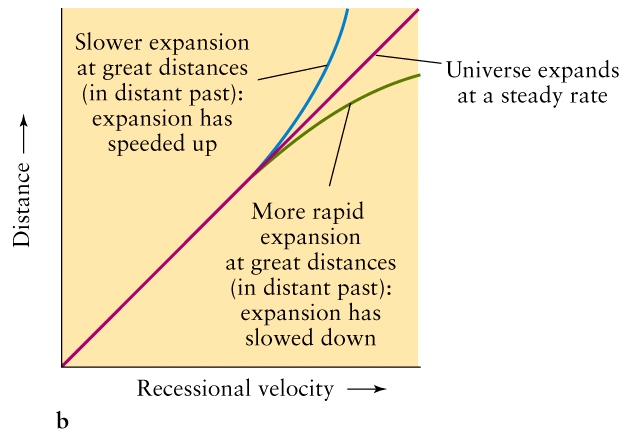## Measurements of the Universe's Acceleration

• Recently astronomers have observed Type Ia supernovae in order to use them as a standard candle to find the distances to the galaxies where the supernovae occur.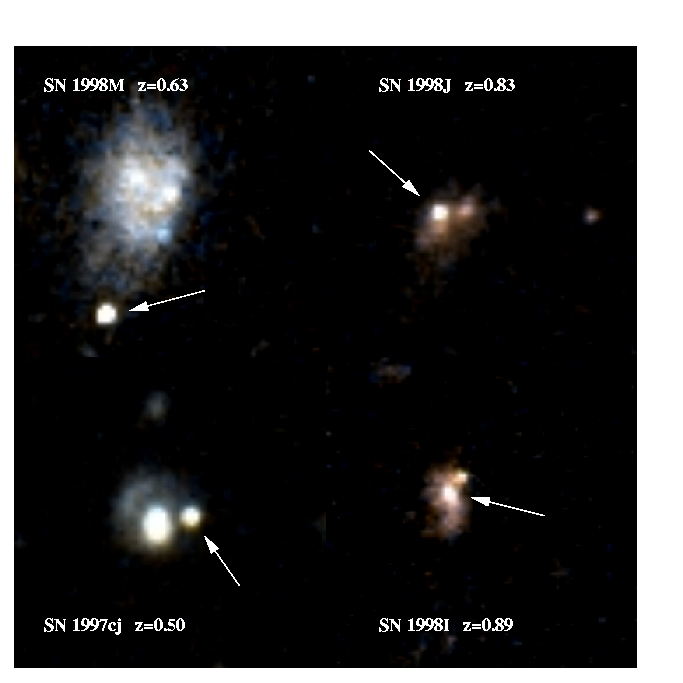## Evidence for Acceleration from Type Ia Supernovae

• The astronomers plotted (M-m) versus redshift (z), which is equivalent to plotting distance versus velocity.
• The data favours a universe whose expansion is accelerating so that the expansion speed increases with time!
• This is opposite to what was expected!
• How the expansion changes depends on the matter content of the Universe, and what type of matter dominates. Einstein equations give us the deceleration parameter

q = &Omegarad + 1/2 &Omegam - &Omega&Lambda
when q > 0 expansion decelerates, while it accelerates if q < 0
• Neglecting &Omegarad, we conclude that Universe was decelerating while &Omegam > 2 &Omega&Lambda and accelerating afterwards.
Note, in this formula we consider all &Omega's as functions of time, not just the values at the present moment. For example &Omegam=&rhom(t)/&rhocrit(t), etc. You can see that to find whether Universe accelerates or not at a particular redshift, one does not need to know how critical density changes with time.
• Note that ordinary matter always causes deceleration. One need an unusual type of energy which density does not decrease with expansion to have acceleration ! This is very strong argument for existenceof "dark energy"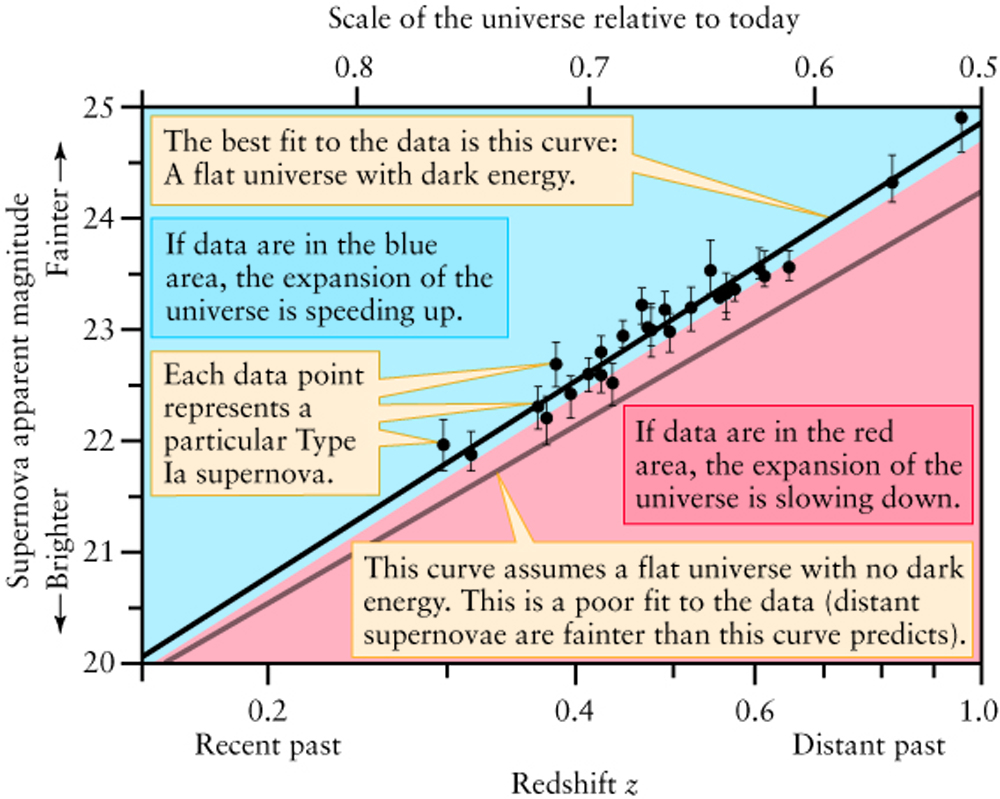## Einstein's Theory of General Relativity

• Einstein's theory of general relativity is the description of gravity which keeps the speed of light as the ultimate speed limit for all information, including gravity itself.
• Einstein solved his equations for a simple model of the universe which has a uniform distribution of galaxies.
• He found that if he placed all the galaxies at rest at constant separation from each other, they would all collapse to a point due to their mutual gravitational attraction.
• If all the galaxies were given an initial velocity so that they were all moving away from each other, it was possible to find solutions which did not collapse, however, no static solution was possible. This was proven by Friedman.

## The Cosmological Constant

• Einstein was very unhappy about the expanding universe solutions to his equations.
• Einstein derived the equations before Hubble and Humason discovered the redshift-distance relation which can imply that the universe expands.
• Einstein thought that the universe should be static and unchanging.
• He realised that the only way to get a static universe was to throw an extra constant into his equations.
• This extra constant is called the Cosmological Constant.
• The cosmological constant has the effect of providing an outwards force sort of like "anti-gravity".
• If the "anti-gravity" effect is chosen large enough to cancel the gravitational attraction of the galaxies, the universe can be static.
• Later when Hubble's law was discovered Einstein discarded the constant.
• Today, we introduce the constant back, however the value of the constant must be much larger than Einstein thought it should be.
• The preferred view is that cosmological constant is an contribution of an unusual form of energy (dubbed "dark").
• If a cosmological constant exists, it has the effect of accelerating the expansion of the universe so that galaxies speed up in the future instead of slowing down.
• If we can measure both the density of matter and the cosmological constant, we can determine the fate of the universe.
• The data from Type Ia supernovae favours the existence of a cosmological constant and a low matter density.
• Their data suggests that the universe will expand forever.
• The physical reason for the existence of the cosmological constant is still unknown.
• An important area of present-day research in theoretical astrophysics is on physical methods for creating a cosmological constant.

## Future of the Universe

• There are three types of solutions to Einstein's cosmological equations.
• They can be understood by analogy with a universe with only two galaxies with equal masses M which are thrown in opposite directions from each other.
1. If the two galaxies are given a large enough initial speed (away from each other) that is larger than the escape velocity, the galaxies continue to move away from each other forever. This type of solution is called unbounded.
2. If the initial speed is lower than the escape velocity, the galaxies move out to some maximum separation and then return to each other so that they come closer to each other. A universe such as this ends in a Big Crunch. This type of universe is called bounded.
3. If the initial speed is exactly the escape velocity, then the galaxies continue to move apart from each other for all time. This is a special solution called the marginally bounded.
• The distance between galaxies for the three types of solutions are shown.
• If there was no "cosmological constant", open and flat Universes would expand forever, while closed Universe will end in big crunch. Geometry would have determined the future fate.
• However, once Universe become "cosmological constant dominated" it will expand with acceleration forever.
• Unless "dark energy" decay somehow in another form of matter.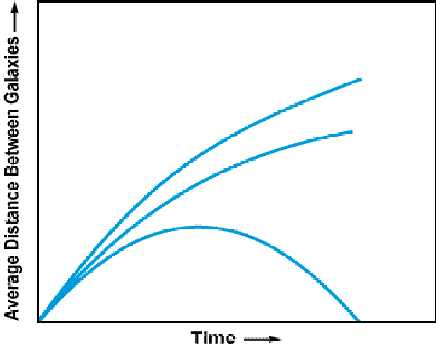## Spatial Topology

• If the geometry of the universe is closed, Einstein's equations do not tell you what the topology is.
• Topology tells you how points on a surface are connected.
• For 2-dimensional surfaces we can classify the types of topology by the number of holes.
 The simplest topology is a sphere which has no holes.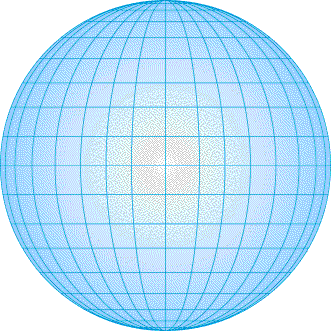The next simplest topology is a torus, which is the surface of a donut.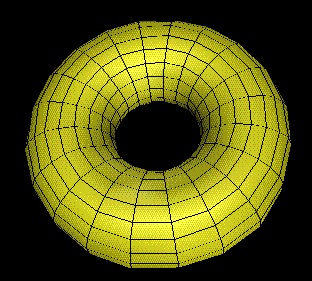• While it may seem unlikely, the universe could have a complicated torus-like topology.
• If so, we would expect patterns in the sky to repeat in regular ways.
• A different way to view a torus is as a square where opposite sides have been glued together.
• A neat way to visualize a torus can be found through some simple games at http://www.geometrygames.org/TorusGames/html/Maze.html

Next lecture: The Cosmic Microwave Background